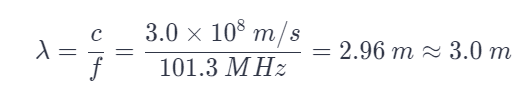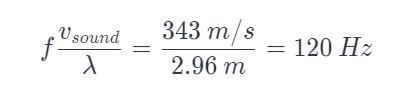# An FM Radio Station Broadcasts at a Frequency of 101.3 MHz. What is The Wavelength?

We thoroughly check each answer to a question to provide you with the most correct answers. Found a mistake? Let us know about it through the REPORT button at the bottom of the page.

A) An FM radio station broadcasts at a frequency of 101.3 MHz. What is the wavelength (m)?

B) What is the frequency (Hz) of a sound source that produces the same wavelength in 20 C air?

A) λ = 2.96 m

B) f = 116 Hz

## Explanation

For part A)

The wavelength is calculated as:For part B)

The speed of sound in air at 20 °C is given as 343 m/s. Hence, the frequency is calculated as: